# ACT Math - Polynomials and Quadratics: Help and Review Chapter Exam

Exam Instructions:

Choose your answers to the questions and click 'Next' to see the next set of questions. You can skip questions if you would like and come back to them later with the yellow "Go To First Skipped Question" button. When you have completed the practice exam, a green submit button will appear. Click it to see your results. Good luck!

### Page 1

#### Question 1 1. What is the b-value of the quadratic equation below?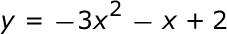#### Question 4 4. Find the solution to the equation below.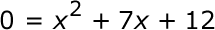#### Question 5 5. Use the quadratic formula to find the solutions to the equation below.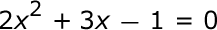### Page 2

#### Question 6 6. Rewrite the following equation in vertex form.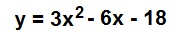#### Question 7 7. Solve the following equation.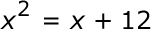#### Question 10 10. Find the value for c that must be added to both sides, and rewrite the equation in the vertex form.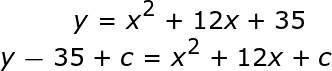### Page 3

#### Question 11 11. In order to complete the square on the following equation, what value would you add to both sides?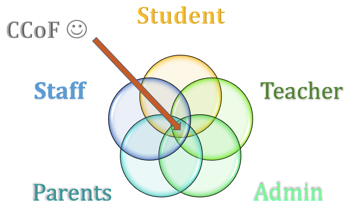#### Question 12 12. Find the roots of the equation.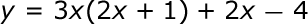#### Question 15 15. Which of the following are the solutions to the equation below?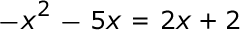### Page 4

#### Question 16 16. What would the first step be in completing the square of the following equation?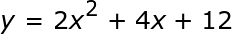#### Question 19 19. Find the solution to the equation below.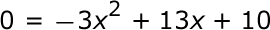### Page 5

#### Question 21 21. If the equation below told you a ball was 'h' feet in the air after 't' seconds, what would be the first step to finding out when it hit the ground?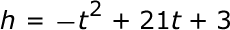#### Question 23 23. Rewrite the quadratic below in standard form: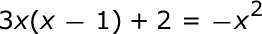### Page 6

#### ACT Math - Polynomials and Quadratics: Help and Review Chapter Exam Instructions

Choose your answers to the questions and click 'Next' to see the next set of questions. You can skip questions if you would like and come back to them later with the yellow "Go To First Skipped Question" button. When you have completed the practice exam, a green submit button will appear. Click it to see your results. Good luck!

Support